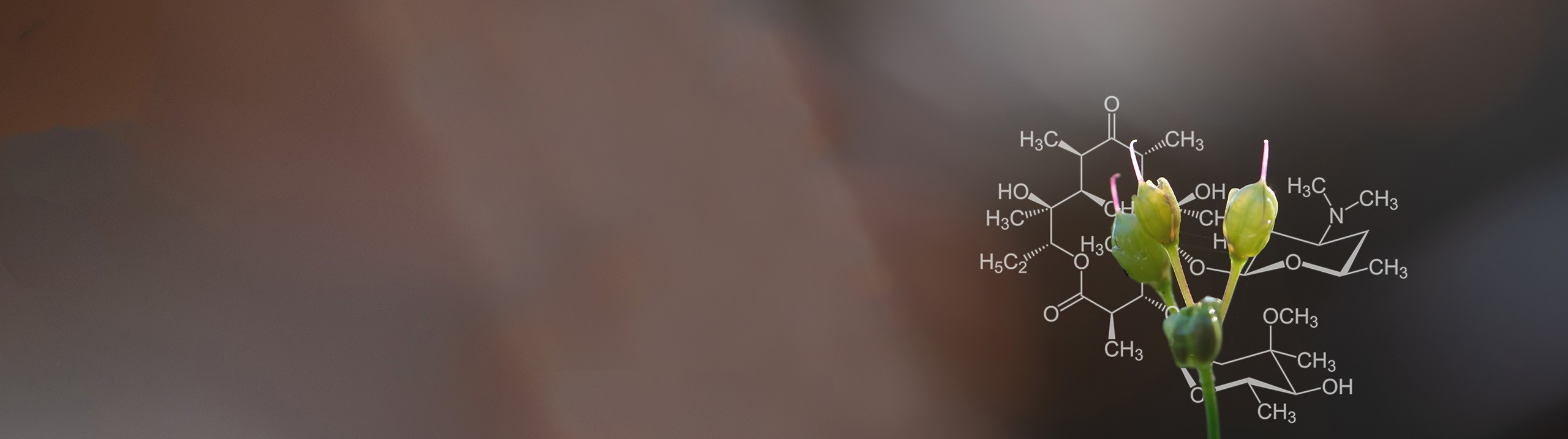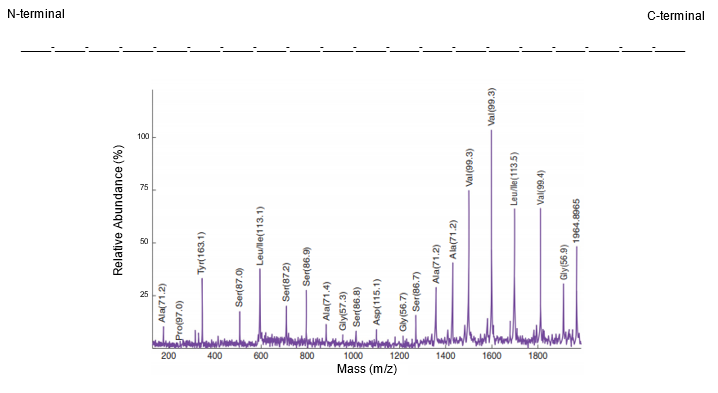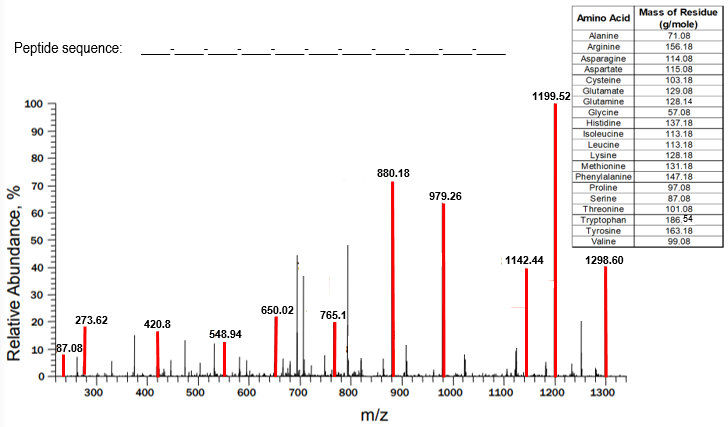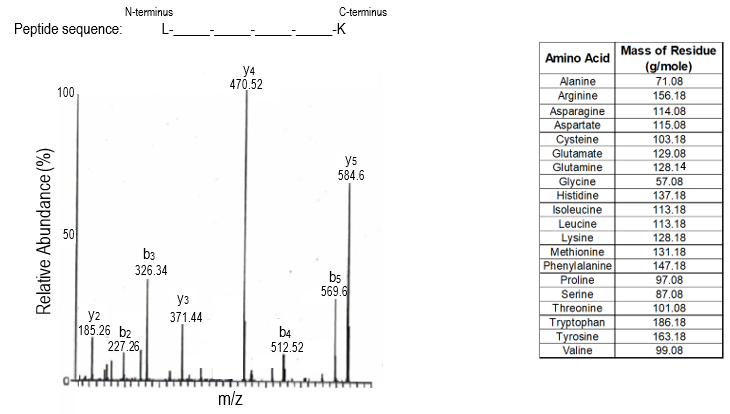Start typing, then use the up and down arrows to select an option from the list.# Biochemistry

Learn the toughest concepts covered in Biochemistry with step-by-step video tutorials and practice problems by world-class tutors

5. Protein Techniques
1

#### concept

Mass Spectrum10m
Play a video:
So now that we've reviewed the basics of mass spectrometry in this video, we're going to talk about a mass spectrum. So a mass spectrum is literally just a plot of the mass spectrometry data or the mass spec data and really just plots the relative abundance on the Y axis of the plot and the MZ ratio on the X axis of the plot. And so if we take a look at our example down below on the left hand side, what we have is a mass spectrum being shown where, indeed, on the y axis, we have the relative abundance. And on the X axis we have the MZ ratio. And throughout our mass spectrum, we have a bunch of these different peaks being shown. And we know from our previous lesson video that most of these peaks correspond with smaller peptide fragments that result from cleavage of our original peptide at Onley. One peptide bond. Now, later, in our course, we're gonna talk more details about how a peptide actually fragments during mass spectrometry. But before we get there, I want you guys to know that a mass spectrum can actually be analyzed to reveal the primary protein structure and recall that the primary protein structure is the amino acid composition, or the number and types of amino acids, as well as the amino acid sequence or the order of amino acids from the N terminal end of a protein to the C terminal end of a protein. And so the way that amino acids are identified in a mass spectrum is by looking at the MZ ratio differences between the appropriate sets of peaks. And so let's take a look at our example down below to clear up this idea of how a mass spectrum can potentially be analyzed to reveal the primary protein structure and so notice that above our mass spectrum, what we have is a Penta peptide being shown or a peptide with five amino acid residues represented by these five different squares. And we know that in general, proteins air considered from their end terminal ends to their C terminal ends. And we also know that most of the peptide molecules in mass spectrometry are going to be fragmented at Onley one peptide bond. But it is possible for some of the molecules of our peptide to get away with, not fragmenting at all. And so, if our peptide does not fragment, that means that all of the peptide bonds are going to remain intact and all of the amino acid residues are going to remain intact as a single unit. And this single unit is gonna have its largest possible mass. And the largest possible mass has the largest MZ ratios, and the largest MZ ratios show up furthest to the right of our spectrum. And so this peak here in light blue actually represents our unframed minted Penta peptide. And so the reason that this peak is blue is because the leading and terminal residue is also blue. Now, even though some of the peptide molecules Congar Oh, unframed minted we know again that most of the peptide molecules and mass spectrometry are going to be fragmented at Onley one peptide bond. So let's imagine that this peptide bond here is being fragmented. Well, essentially, what's gonna happen is this residue here on the end is gonna be cleaved off, and this fragment here is going to be detected by the mass spectrum. And so this fragment here is gonna have the next largest MZ ratio, and it's going to correspond with this highlighted peak shown there. And so really, the only difference between this yellow peak here and the original blue peak of the unframed minted Penta peptide is the the mass of this one residue here. And so if we take the mass difference the Delta Mass between these two peaks right here, which comes out to 99.8 that will give us the mass of this one residue that what's cleaved off. And so, looking at our chart over here we have amino acids listed on the left and then the molecular weight of the residue and grand per mole listed on the right and noticed that the difference in mass between these two peaks came out to 99. And analyzing all of these molecular weights, notice that it matches the molecular weight of veiling because most of the amino acids have unique molecular weights, and so of molecular weight of 99.8 corresponds with a veiling amino acid residue. And so that means that this blue residue appear is a veiling residue, and we were able to obtain that just by imagining the cleavage of this one peptide bond. Now, let's imagine that not this peptide bond, but perhaps a different peptide bond is Cleave. So let's imagine that this peptide bonds cleave this time. Well, essentially, what's gonna happen is this entire fragment here is gonna be cleaved off, and the Onley part that's gonna be remaining here is this fragment right here, which corresponds with next largest MZ ratio peak, which would be this green peak right here. And so essentially, the only difference between, uh, this Green Peak and this yellow peak is the loss of just this one. Uh, this one residue here. The only difference between, uh, these two peaks is the loss of just this one pink peak here. And so, essentially, what we can see is that the difference in Masses 137.18 and looking at our chart notice that it matches with the molecular mass of a spare gene 37.18 And so we can put a spare jeans, three letter code here, and a spare gene would be this residue. And so you can see here how we're literally taking the differences in MZ ratio between the peaks to identify the amino acids because most of the amino acids have unique molecular weights. And so you can kind of see how the pattern is working for this. So if we were to continue this essentially, what we would do is cleave this peptide bond. We would cleave off this fragment and we would get this fragment here showing up as this peak right here. We would take the difference between these two peaks. And the difference between these two peaks comes out to 71.8 which matches with Alan in 71.8 And so, essentially, what we can do is put in Allan in here and up here. So we're gonna continue Thio, analyze all of these residues. And of course, if we were to cleave this last peptide bond here, we would fragment we would release this fragment. This one would be detected right here. And so we can take the differences between these two peaks to reveal this amino acid and that comes out toe 114.8 which is a history so we could put history in here and history and up here And you guys were getting the pattern of this. So essentially, what you can see is that this last residue here is gonna be all by itself as a single peak. And so it's mass is literally gonna be determined by the difference between the zeros of mark on the X axis and the peak itself. And so that comes out to a difference in Mass of 57.8 which matches with the mass of a glazing residue here so we can put glass in here and glazing as our last residue. So now what you might may or may not have noticed is that literally are mass spectrum is revealing the sequence of our peptide backwards. So notice that we're analyzing our mass spectrum from the right to the left in order to reveal our sequence from the end terminal end to the C terminal end. And so that's actually one of the major points that I want you guys to take from this video. And so what I want you guys to know as that mass spectra are typically analyzed from the right to the left of the spectrum to reveal the peptide sequence. And so again, we're starting here. It starts at veiling to reveal the internal sequence, and then we continue to read it from the right to the left to reveal our sequence from internal to see terminal. So the reason for why we need to do that? We're actually going to talk about another lesson video a little bit later in our course and again, for now, I just want you guys to know that we typically analyze spectrums from the right to the left. Now one of the actual limitations of mass spectrometry is that it actually struggles to differentiate. Losing from is a losing, and the reason for that is because losing and is a losing our ice summers of one another. And that means that they have the same exact chemical. Adams just arranged in a different format, and so because they have the same chemical Adams, they have the same exact mass. And so when we have a mass difference, it could correspond to either isil oozing or losing, and mass spectrometry cannot differentiate between them. And so that's actual limitation of mass spectrometry. So this here concludes our initial introduction of a mass spectrum and again in our next lesson Video will be able to talk Maura about how a peptide actually fragments during mass spectrometry. But before we get there, let's get a little bit of practice, so I'll see you guys in our practice video.
2
Problem

Use the mass spectrum below to determine the sequence of the peptide.3

#### concept

Mass Spectrum9m
Play a video:
4
Problem

Upon fragmentation of a peptide bond during mass spectrometry, what ions can be detected on the spectrum?

5
Problem

Use the mass spectrum below & the indicated y-ion peaks (red) to reveal the sequence of the peptide.6
Problem

In your mass-spectrometry of a pure protein with an m/z of 1,582, you found peaks of y ions with the following m/z ratios of 1,582, 1396 and 1283. The mass in Daltons for the possible relevant amino acids are provided: Y (163), N (114), W (186), D (115), G (57), L (113) and M (131). From this data, it is obvious that the C-terminal amino acid residue of the 1,582 fragment is:

7
Problem

Use the mass spectrum below & the provided chart with amino acid masses to determine the sequence of a hexapeptide (6 amino acid residues). In the mass spectrum, y ion peaks are indicated with “y” while b ion peaks are indicated with “b.” The N-terminal residue is given as Leu and the C-terminal residue is given as Lys. Determine the remaining amino acid sequence using either the y ions or the b ions.